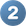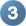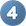# DArTView¶

DArTView is a KDXplore plugin for marker data curation via metadata filtering. DArTView enables users to overcome tedious manual calculation of marker data that is common when using spreadsheet applications. This may be done with the following steps:

1. Creating a formula for a statistic (e.g. Marker Call Rate);

2. Extending the formula to the entire dataset;

3. Sorting data via calculated statistics;

4. Visualising calculated statistics (e.g. via scatter plot);

5. Subsetting dataset based on the determined threshold; and

6. Repeating steps 1-4 to look at the effect on other statistical distributions.

This user guide provides an overview of DArTView, as well as functions such as those listed above. If you would like access to DArTView then see the KDXplore Getting Started page.

Note

## Supported Formats¶

DArTView supports multiple nucleotide genotypic data types of DArT and Hapmap marker data formats:

DArT

• DArT Counts

• DArT SNP Two Row

• DArT Silico SNP

• DArT Silico Counts

• DArT SNP One Row

Hapmap

• Two Letter

• SSR

• Codominant

• Dominant

Note

These formats will be referred to as marker data throughout this user guide.

## DArTView Main Window¶

The DArTView Main window displays an opened file, as well as filters and graphs that have been applied. See the below image and table for more information about the sections of the DArTView Main window:

Panels of the DArTView Main Window

Item

UI Element

DescriptionLists metadata that is organised into markers/samples with subsections numeric/non-numeric each. Checked metadata are displayed as a graphs in the Graphs panel.Filters Panel

Lists all active/inactive filters that have been added to the file and provides options for managing these filters.Graphs Panel

Displays scatter and histograms plots based on the filters applied to a file.

Data can be viewed after it is loaded from a marker data file. The options for viewing data are outlined in the image and table below:

Viewing Data

Item

UI Element

DescriptionReport Counts

The total number of markers and samples are displayed here.Select either the Markers tab or the Samples tab to view metadata on markers or samples from the report. The Numeric tab and Non-Numeric tab allows the user to view numeric and non-numeric metadata.Stats Button

The Metadata Panel in the above image displays only basic metadata stats. Select the Stats button to choose the all stats option which displays all metadata stats available.Genotypic Data Map button

This button will open a window called the Genotypic Data map where data can be graphed. See the section below for more information.This panel displays metadata according to what tabs the user has selected to view. Select the checkbox or double-click on any of the rows to display further information on the metadata. This is demonstrated in the image below where the callrate is selected and displayed.

DArTView provides both numeric and non-numeric summary statistics for marker data files. The below tables lists these statistics and provides descriptions for each one:

Numeric Summary Statistics

Statistic

Description

Min

The minimum value for this metadata statistic.

Mean

The mean value for this metadata statistic.

Max

The maximum value for this metadata statistic.

Extended: Q1

First interquartile range. The middle value in the first half of the rank-order data set.

Extended: Median

Second interquartile range. The middle value of the rank-order data set.

Extended: Q3

Third interquartile range. The middle value in the second half of the rank-order data set.

Extended: Variance

The sample variance of the statistic: $$\frac{1}{N-1}\sum_i^N (\overline{X} - X_i)^2$$

Extended: Std Dev

The sample standard deviation of the statistic: $$\sqrt{\frac{1}{N-1}\sum_i^N (\overline{X} - X_i)^2}$$

Extended: Std Err

The sample standard deviation of the statistic: $$\frac{\sqrt{\frac{1}{N-1}\sum_i^N (\overline{X} - X_i)^2}}{\sqrt{N}}$$

Non-Numeric Summary Statistics

Statistic

Description

Values

Displays some entries of the metadata

Source

Description

Calculated

Metadata has been calculated using DArTView’s internal set of calculators for this data format.

Report

Metadata was imported from a report.

Imported